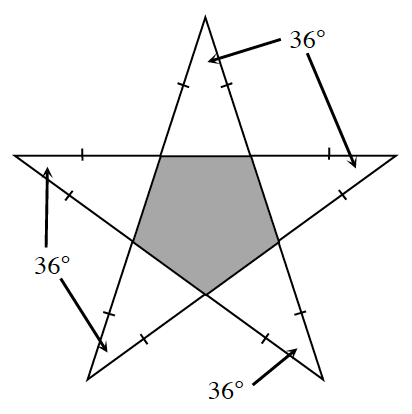### Home > CCG > Chapter Ch10 > Lesson 10.2.2 > Problem10-82

10-82.

After doing well on a test, Althea’s teacher placed a gold star on her paper. When Althea examined the star closely, she realized that it was really a regular pentagon surrounded by $5$ isosceles triangles, as shown in the diagram below. If the star has the angle measurements shown in the diagram, find the sum of the angles inside the shaded pentagon. Show all work.What do the tick marks on the sides of the triangles mean?
How can you use these to find the measures of the other angles in the triangles?

Once you have the measures of the other angles in the triangles, how can you use these measures to find the measures of the angles in the pentagon?